Tableau

# How to create a printable table with multiple pages in Tableau

This isn’t my favourite use of Tableau by any stretch of the imagination, but it’s something that comes up now and again when doing Tableau consulting:

“I’ve got a massive table, which is fine to scroll through online, but I can’t print it. How can I print out this table over multiple pages while keeping all the dashboard formatting and the column headers?”.

My solution to this uses a parameter and a running total calculation using the [Number of Records] field. You can download my workbook from Tableau Public here, and then follow the instructions below.

First of all, let’s create a big old table, something a little like this:

It’s got almost 10,000 rows in it. That’s fine when you’ve got an interactive scroll bar and you’re working with it online, but not so much if you need to create static print outs.

So, the next step is to find a way of making it into pages. What I want to do is put the table on a dashboard, like this:

…and instead of having a scroll bar, I want to fit the data to however many rows fit on the dashboard, and then repeat that dashboard as many times as necessary.

Let’s bring in the AVG([Number of Records]), and switch it to discrete so it functions like a row number where the row number is 1 for each row:

Now let’s add a running total table calculation to it, computing along Table (down). This gives us a dynamic Row ID:

The next step is to create a parameter to select the page number. You’ll need to make it an integer with any allowable value.

Now, we can divide the table into pages. I’ve decided that I’d like to show 25 rows on each page, mostly because that’s an easy number to work with in my head – I know that there’ll be 4 pages for each 100 rows in the data.

We can use the following logic to determine where my 25 row pages start and end:

((RUNNING_SUM(AVG([Number of Records]))-1) / 25) + 1

This is a few more brackets than are technically necessary, but I find that it clarifies the purpose of the calculation. It takes the dynamic Row ID we’ve created, and subtracts 1 from it, so that it goes like 0, 1, 2, 3… instead of 1, 2, 3, 4… and so on. Then, it divides that number by 25, which is the number of rows I want in each table page. Finally, it adds 1 to the whole thing.

This tells us where each page will be:

Why does it subtract 1 and then add 1 again? The first -1 is in order to make sure that all pages have the same number of rows on them. If the Row ID begins on 1, then the first page will always have one row fewer on it, as it’ll take rows 1-24, then the second page will take rows 25-49. Subtracting 1 means that the first page will take rows 0-24, then the second page will take rows 25-49. Then, after dividing the Row IDs by 25, the first page will have a number between 0 and 1. Talking about the first page as page 0 and the second page as page 1 always gets confusing, so I’ve added 1 back on to make it more intuitive.

Now that we’ve got that logic understood, we can create a Page Filter calculated field:

((RUNNING_SUM(AVG([Number of Records]))-1) / 25) + 1 >= [Page Selector]
AND
((RUNNING_SUM(AVG([Number of Records]))-1) / 25) + 1 < [Page Selector] + 1

This filters the table to whichever page you’ve selected in the page selection parameter. So, if you’ve selected page 4, it’ll give you all values where the Row ID divided by the number of rows you want per page is >= 4 and < 5. This corresponds to rows 76-100.

Now that the filter is set up correctly, we can get rid of the first two columns in this table entirely and leave it to work in the background. You can put the new version of your table into a dashboard along with the page selector parameter. Tableau also lets you set the dashboard size to common printer paper sizes, so I’ve set this to A4 portrait:

Now, if you need to print out the entire table in a consistent format, you can cycle through all the pages and print them individually. This will obviously take a lot of time for big tables, and it won’t be a pleasant experience, but it does at least make it possible for you!

StandardTableau

# Standard errors and confidence intervals in Tableau

### tl;dr version

Here’s how to make bar graphs with standard errors and confidence intervals in Tableau. It involves making some calculations yourself, which may or may not differ from Tableau’s built in versions. You can download the workbook showing you how to make the graphs here:
https://public.tableau.com/profile/gwilym#!/vizhome/Standarderrorsandconfidenceintervals/Standarderrorbarsoptions

### Why show variance in your graphs?

Sometimes when you plot values on a graph, you want to show not only the aggregated value, but also the variance or uncertainty around it. Now, before I get into this blog properly, I want to say that I don’t actually recommend plotting bar graphs with error bars or confidence intervals, as it can be misleading. The Bar Bar Plots campaign has far more information on it, but ultimately it’s more honest, and really straightforward, to show the actual data points in Tableau, so why wouldn’t you just do that?

Friends don’t let friends make barplots – solid advice from Page Piccinini.

But in the event that you do need to show simple bars and an indication of uncertainty, you’ve got two main options:

1. Standard errors
2. Confidence intervals

### Introduction to the data

I’m going to use some data I collected during an experiment I ran in 2015. In this experiment, Dutch people learned some Japanese ideophones (vividly descriptive words). But there was a catch – half the words they learned were with the real meanings (e.g. fuwafuwa, which means “fluffy”, and they learned that it meant “pluizig”), and half the words they learned were with the opposite meanings (e.g. debudebu, which means “fat”, but they learned that it meant “dun”, or “thin”). Then they did a quick test to see if they remembered the word associations correctly. You can read more about that here, if you like.

All the following graphs in this blog have been created in this workbook on Tableau Public. Please feel free to download and explore how it’s all made!

Here’s a simple bar graph of the results. For the words they learned with their real meanings, people answered correctly in the test round 86.7% of the time. But when tested on the words they learned with their opposite meanings, people answered correctly only 71.3% of the time.

But this hides the variation in the data. Sure, the average in each condition (and the difference between them) is what I care about, but with simple bar graphs, it’s easy to forget that lots of individual people are below and above the average in each condition. You can see that variation here:

Also, these are averages taken from a sample. I can’t go to a conference and say, “hey everybody, I’ve done the research and Dutch undergrads get 86.7% correct in the real condition and only 71.3% in the opposite condition”… well, I could, but it would be misleading. I can’t guarantee that these results are definitely in line with what the entire population of Dutch undergrads would get if I somehow managed to test all of them, so I need to make some kind of statement about the uncertainty of that result. I can do this with standard errors or confidence intervals.

### Standard errors

Let’s start with standard errors. The standard error of the mean is essentially a way of saying how uncertain you are about the mean based on the size of your sample by estimating the standard deviation of the whole population. The wikipedia article on standard errors is pretty good.

The first step is to create a field for the standard error. This is the standard deviation of the scores per condition, divided by the square root of the number of participants:

STDEV([Correct])
/
SQRT(COUNTD([Participant]))

You’ll notice I’ve also got fields for the sample standard deviation and the not sample standard deviation. This is from when I was playing around with different calculations for the standard deviation of the sample vs. the standard deviation of the population. I’m not going to go into it in this blog, but here’s a really nice explainer here, and you can download the workbook to investigate further. In summary, it looks like Tableau’s native STDEV() function uses the formula for the corrected sample standard deviation by default, rather than the population standard deviation. This is pretty nice, it feels like a safer assumption to make. Cheers, Tableau.

Now that we’ve got the standard error, we can create new fields for our upper and lower standard error limits like this:

AVG([Correct]) – [SE]
and
AVG([Correct]) + [SE]

So, now we can create some nice standard error bars. This uses a combination of measure names/values and dual axes, so it’s a little bit complicated. Firstly, create your simple bars for the correct % per condition:

Now, drag the lower standard error field onto rows to create a separate graph. Drag the upper standard error field onto the same axis of that new graph to set up a measure names/measure values situation:

Now, switch the measure values mark type to line, and drag measure values from columns and drop it on the path card:

All you have to do now is create a dual axis graph, synchronise the axes, and remove condition from colour on the standard error lines:

Great! We’ve now got bar graphs with standard error bars. I mean, I still don’t recommend doing this, but it’s a common request.

### Confidence intervals

Now, let’s have a look at confidence intervals. They are a range around your sample mean which tell you that, if you repeated the same study over and over, X% (usually 95%) of confidence intervals from future studies will contain the true population mean. They’re hard to explain (there’s a good blog here), but easy to see.

In Tableau, confidence intervals are really straightforward. You can plot your data points, go to the analytics pane, and bring in an “average with 95% CI” reference line, which creates a reference band around the average:

Nice. This is exactly how I’d like to visualise my experimental data! You can see the average per condition, the confidence intervals, and the underlying participant data.

Quick disclaimer: because I’m looking at percentages here, this is a proportion rather than a hard and fast value, so I shouldn’t actually be using confidence intervals at all… but if we pretend that the 86.7% value is actually an average 0.867 value of something like my participants taking 0.867 seconds taken to respond, or young children being 0.867 metres tall at a certain age, or 0.867 kg lost for each week under a new diet plan, then it’s okay. I’m just going to keep going with my percentages, but please bear this in mind.

However, if your journal insists on old school bar graphs, Tableau’s built in average with 95% CI reference band won’t work. Well, technically it will, it’s just that it’ll show you this:

Because we’ve had to take Participant off detail in order to show an aggregation across participants, the reference band doesn’t know how to compute it, and it assumes that there’s just one data point.

One way around this would to built a dual axis graph. Keep the bars with just condition on colour, and create another axis. Add participant to detail, and set the mark type to circle. Make the circles as small as possible and completely transparent, hit dual axis, synchronise axes, and voila. Now you can have an average with 95% CI reference band again.

The downside is that this is pretty ugly. The reference line/band is way outside the edges of the bars, and it just doesn’t have that standard look that you’re used to. What we actually want is something like our standard error lines from earlier, but with confidence intervals.

The good news is that we can do it! But we’ll have to move away from Tableau’s built in confidence intervals, and create our own calculation, just like we did with standard errors.

The first step is to use the standard error field we made earlier to calculate the confidence intervals. When you look up how to calculate confidence intervals, you’ll probably find something saying that 95% confidence intervals are calculated by taking the mean, and adding/subtracting 1.96 multiplied by the standard error. This 1.96 figure is from the Z distribution, which tells you that 95% of normally distributed data is within 1.96 standard deviations of the mean. And because this is a sample of a population, we multiply that 1.96 by the standard error to get our confidence intervals. Here’s another great blog which breaks it all down.

So, we can create separate fields for our upper and lower confidence interval limits like this:

AVG([Correct]) – (1.96 * [SE])
and
AVG([Correct]) + (1.96 * [SE])

Once we’ve done that, we can build our graphs. This is the same technique as the standard error bars earlier. Create the measure names/values and dual axis graph with measure names on the line path, and you’ll get the same kind of graph, but now showing confidence intervals instead of standard errors:

Excellent! We’ve now got our 95% confidence intervals… or do we?

### Confidence intervals, pt.2 – what’s going on?

Some of the more statistically minded of you may have been yelling at the screen when I used the 1.96 value from the Z distribution to calculate my confidence intervals. You see, confidence intervals shouldn’t always simply use the Z distribution, even though that’s the standard formula you’ll find when looking up the definition of confidence intervals. Rather, when you’ve got a small sample, which is generally defined as under 30, you should use the T distribution because the size of the sample may skew the normality of the sample. Again, there’s a lot of good information here.

I started investigating this when I noticed that Tableau’s average with 95% confidence interval calculations were different from my manually calculated ones. Have a look at this comparison – you’ll notice that the confidence interval values are slightly different:

I started playing around with the Z/T value in the confidence interval calculation by making it parameter-driven, and I found that Tableau’s confidence interval calculation seemed to use a number like 2.048 rather than 1.96:

This is because Tableau’s confidence interval calculation is using the T distribution rather than the Z distribution. You can find the appropriate T values to use based on your degrees of freedom (which is your sample size minus one) in Appendix B.2 of this very useful pdf (there’s also a table set to 4dp instead of 3dp here). In my case, I’ve got 29 participants, so the degrees of freedom is 28, and the lookup table shows that the relevant T value for a 95% confidence interval is 2.048, so I can put that in my confidence interval calculations. It also looks like Tableau’s confidence intervals are calculated on a more precise number than 2.048, which suggests that the back end is calculating it directly from the T distribution rather than using the fairly common approach of looking it up in a table where everything is rounded to three decimal places. That’s pretty nice too.

My next step was to check whether Tableau switches between the T and Z distributions based on sample size. So, I duplicated my data and fudged the [correct] field by a random number to create a sample of 58 participants. With 58 participants, it’s fine to use the Z distribution to calculate 95% confidence intervals. But even then, it looks like Tableau is using the T distribution – when I set my parameter to 2.0025 using the slightly-more-precise values in the T table here, you can see that the confidence intervals using T values, not Z values, match Tableau’s calculations:

This is pretty good as well, I think. As your sample size increases, the T distribution starts to match the Z distribution more and more closely anyway. Notice how, with 29 participants, the T value was 2.0484, and with 58 participants, it was 2.0025. This is getting closer and closer to 1.96. At 200 participants, the T value would be 1.9719. Overstating the confidence intervals by using the T distribution is safer default behaviour than accidentally understating them by using the Z distribution.

So, to conclude, I’ve found out the following about confidence intervals in Tableau:

1. They’re based on standard errors which use the corrected sample standard deviation (and Tableau’s STDEV() function returns the corrected sample standard deviation as well).
2. They’re based on the T distribution regardless of your sample size.

Again, I’ve published the workbook containing my demo graphs and my standard deviation and T vs. Z explorations here: https://public.tableau.com/profile/gwilym#!/vizhome/Standarderrorsandconfidenceintervals/Standarderrorbarsoptions

One final word of thanks to my colleague David for helping me out with some of the troubleshooting!

Standard

# Donut charts and dynamic reference banding

Donut charts aren’t everybody’s cup of tea, but I quite like them for showing a percentage against a total which has to be 100%. Things like the percentage of tickets answered within an hour, or an industrial test pass rate as a percentage, or an on time percentage.

The problem is that percentages often come with targets. If you’re measuring a rate, you’re probably measuring it to check that you’re on target. For example, if you’ve got 19.8% of tickets being answered within an hour, you’ve probably also got a target of 15% or 20% or something, and you’d probably want to show that on your donut chart for context, like this:

In Tableau, you can’t do that, not without creating some pretty filthy trigonometric calculations. But I’ve recently found a workaround which I rather like, which I’ll explain in this blog. You can download the supporting workbook from Tableau Public here.

I’ve used Superstore, which isn’t too ideal for percentages and targets, but hey, it’s something everybody uses. Let’s say you’re the head of sales for California. You know you’re a big market, and you want to keep it that way – you want 15% of all of Superstore’s sales to be in California.

You can create donut charts showing this percentage easily by creating two fields. One called [California Sales], which is:
IF [State] = “California” THEN [Sales] END

The other would be [Rest of US Sales], which is:
SUM([Sales]) – SUM([California Sales])

And you’d put it on a donut chart with those two fields as the two measure values, then put measure names on colour, and split it out by category to get something like this:

Sadly, we can’t put a reference line at the 15% mark to show the target. Not easily, at least. But what we can do is to play around with the colours. If the percentage is above the target, we could show the percentage up to the target in yellow, and then the overperformance in green, like so:

And if we adjust the target higher, we could show the percentage up to the actual percentage in yellow, and then the underperformance in red, like so:

This is a little complicated. It requires a few extra calculations; [California Sales Percentage], [Target Distance], [California Sales Base], [Rest of Sales], [California Sales Over], and [California Sales Under]. Let’s go through the logic one by one.

[California Sales Percentage]
In this calculation, you take the existing [California Sales] field that you’ve made, and found out what that is as a percentage of all sales. It’s simply:
SUM([California Sales]) / SUM([Sales])

[Target Distance]
This is how far from the target the California Sales Percentage is. I’ve used [Target] as a parameter to make it adjustable, but you could also hardcode it. It’s simply the California Sales Percentage minus the target; so, if you’ve got an actual % of 21%, and your target is 15%, then the Target Distance will be 6%. It’s simply:
[California Sales Percentage] – [Target]

[California Sales Base]
This calculation will be what’s in yellow in the donut. If your California Sales Percentage is above the target, then you’ll want it to be yellow up to the target, and then green above that, so this base field will simply be the target. If your California Sales Percentage is below the target, then you’ll want it to be yellow up to the actual sales percentage, and then red for the space between the percentage and the target. So, you can calculate it like this:
IF [Target Distance] > 0 THEN ([Target] * SUM([Sales]))
ELSE SUM([California Sales]) END

[Rest of Sales]
This is the bit in grey. If your California Sales Percentage is above the target, then you’ll want it to be grey from the actual sales up to 100%. If your California Sales Percentage is below the target, then you’ll want it to be grey from the target value up to 100%. That can be calculated like this:
IF [Target Distance] < 0 THEN
SUM([Sales]) – ([Target] * SUM([Sales]))
ELSEIF [Target Distance] > 0 THEN
SUM([Sales]) – SUM([California Sales])
END

[California Sales Over]
This is the bit in green. If your California Sales Percentage is above the target, then you’ll want it to be green between the target and the actual sales percentage. If it’s below target, you don’t want it to show up at all, so set it to zero like this:
IF [Target Distance] > 0 THEN
SUM([California Sales]) – ([Target] * SUM([Sales]))
ELSE 0 END

[California Sales Under]
Finally, this is the bit in red. If your California Sales Percentage is below the target, then you’ll want it to be red between the actual sales percentage and the target. If it’s above target, you don’t want it to show up at all, so set it to zero like this:
IF [Target Distance] < 0 THEN
([Target] * SUM([Sales]))-SUM([California Sales])
ELSE 0 END

Okay! Now we’re ready to build our donuts. This is the easy bit.

Build out your donuts like normal, like this:

Now, instead of the current two measure values, we’ll want all four of the colour ones:

For this one, I’ve set the target to 20% so that there are examples of categories that are above and below target, all in one view.

And that’s it! It’s not quite a reference line, but it’s a nice way of showing a percentage performance against a target within a donut chart. As a reminder, you can download my workbook here:
https://public.tableau.com/profile/gwilym#!/vizhome/Dynamicreferencebandingondonutcharts/Donutchart-dynamicreferencebanding

Standard# Eurovision Song Contest: a market basket analysis of voting patterns and international relations.

I’ve been doing a lot of market basket analysis at The Information Lab lately. Market basket analysis is a way of looking for things that people buy at the same time (or that people never buy at the same time) in order to spot trends in people’s behaviour. For example, it’s probably obvious that if somebody buys cereal, they’ll probably also buy milk. Or that if somebody buys tofu, they’re not going to be buying sausages. This is a really nice example of how it all works.

Thing is, after a while, using bread and butter or cereal and milk or sausages and tofu as an example gets kinda dry. And talking about Lego shovels and milligram-level accurate scales is sometimes a little unprofessional, even if it is a perfect example of consumer behaviour.

So, I’ve been analysing the Eurovision Song Contest. The jury votes lend themselves pretty well to market basket analysis, because they’re pretty similar to transactions: each country’s jury (or customer) votes for (or buys) ten countries (or items) at a time (in a basket), and the fact that these countries (or items) are a subset of all possible countries (or items) to vote for (or buy) means that you can make the same selection vs. non-selection distinction. And we all know that some countries always vote for some other countries, regardless of how good the song is, which is part of what makes it fun.

I took the historic Eurovision data collected by Stephan Okhuijsen of Datagraver. Then, using Alteryx, I filtered it to all contests from 1993 onwards, because European countries have been relatively consistent since then. I also filtered it to the final only, and to the jury votes only.

I set the minimum support for a rule to 0.01, which is kind of high for a regular market basket analysis using tens of thousands of SKUs in a supermarket, but works fine for such a closed set of possible choices of countries. I also set the minimum confidence to 0.05. That gave me almost 33,000 association rules, of which about 1,600 were one-to-one country mappings.

The full results are in an interactive dashboard here.In the matrix at the bottom, you can see who consistently votes for who, and it’s pretty predictable. Cyprus and Greece, for example, almost always give each other the most possible points. There’s a big love in between Moldova and Romania, and between Turkey and Azerbaijan. The Nordics are a bit too cool to give each other full marks every time, but it’s still a bit of a Scandi circle jerk. Andorra love Spain, although it doesn’t seem like that’s reciprocated. Azerbaijan have never voted for Armenia, funnily enough. And Austria have given Australia full marks twice, which I like to believe is because they were hoping to exploit a poor fuzzy matching process in the background scoring:But market basket analysis shows how countries behave as a group, where we can see how some associations are Europe-wide, and some are just confined to the two countries. For example, some of the Scandi trends are reflected in votes across Europe; if a country, any country, votes for Denmark, they’re also likely to vote for Norway:And surprise, surprise, countries that vote for Ukraine will also vote for Russia:But the Greece/Cyprus love in is special just for them; in fact, if anything, there’s a slightly negative association between them, meaning that if a country votes for Cyprus, they’re slightly less likely to vote for Greece as well:Likewise with Turkey and Azerbaijan. Just because they give each other full points all the time, other European countries don’t link the two together in their voting behaviour at all:Meanwhile, even though Azerbaijan will never give points to Armenia, and Armenia have only ever given one point to Azerbaijan, other European countries are far more optimistic. Maybe they hope that voting for both Armenia and Azerbaijan at Eurovision can resolve the Nagorno-Karabakh dispute. Or maybe they just don’t know anything about the Caucasus region and think they’re the same place, I don’t know.This is quite nice to illustrate, because the market basket analysis allows you to make the distinction; while there are some obvious associations between countries, like how Greece and Cyprus always vote for each other, it shows that those associations aren’t necessarily transferred to other countries’ voting behaviour.

Click through to the interactive version here to explore in more detail. I’m going to be using this in my teaching examples more often.

Standard
Tableau

# Using the modulo function to only show full quarters

I don’t use the modulo function (which is just % in Tableau) very often, but every so often, I find a really handy solution to something which uses it. In this case, it was a neat way to only show full quarters of historical data.

Here’s a view of all sales by segment per quarter in the Superstore data: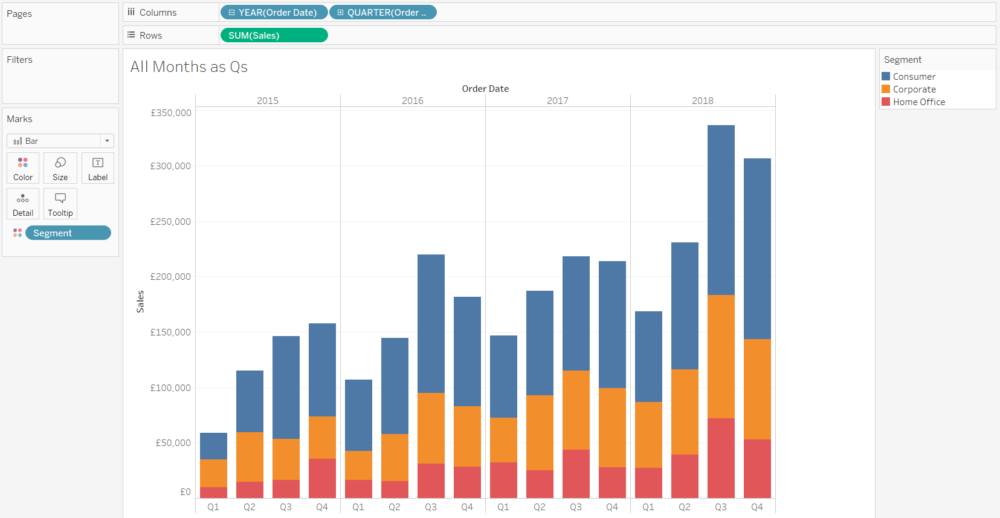Nice and simple, no filters or restrictions – these are the sales by segment per quarter across the whole data set.

But something I often do for clients is create a date parameter so that they can use a dashboard to generate historical reports, or show what the business looked like at a particular point in time. So, here’s a month selection parameter, which allows you to select any particular month in the data: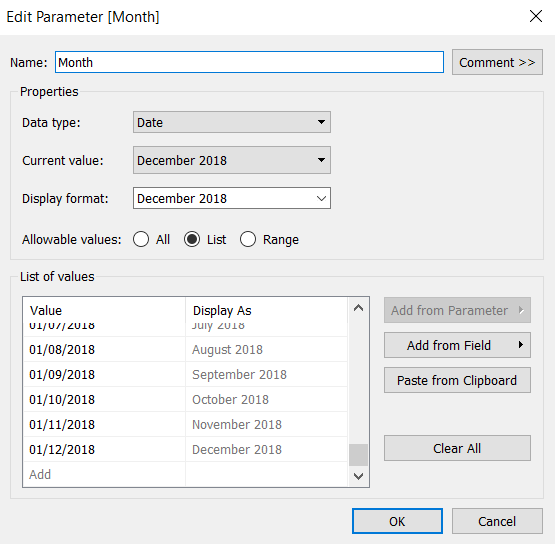…and that can be combined with a Last Twelve Months filter, which will filter the data to the twelve months up to and including the month selected in the parameter. So, if you select November 2018, it’ll show you all the data between 1st December 2017 and 30th November 2018.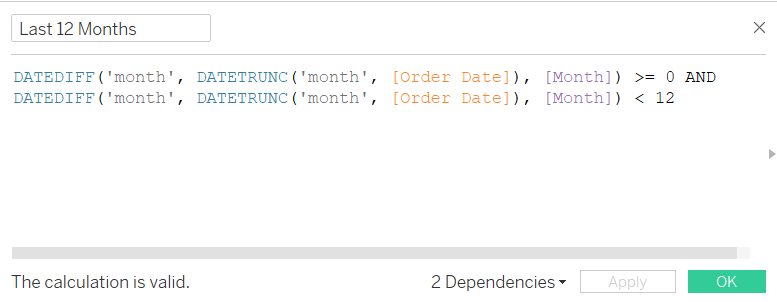So, let’s have a look at what happens when we apply our Last Twelve Months filter to our sales by segment per quarter view: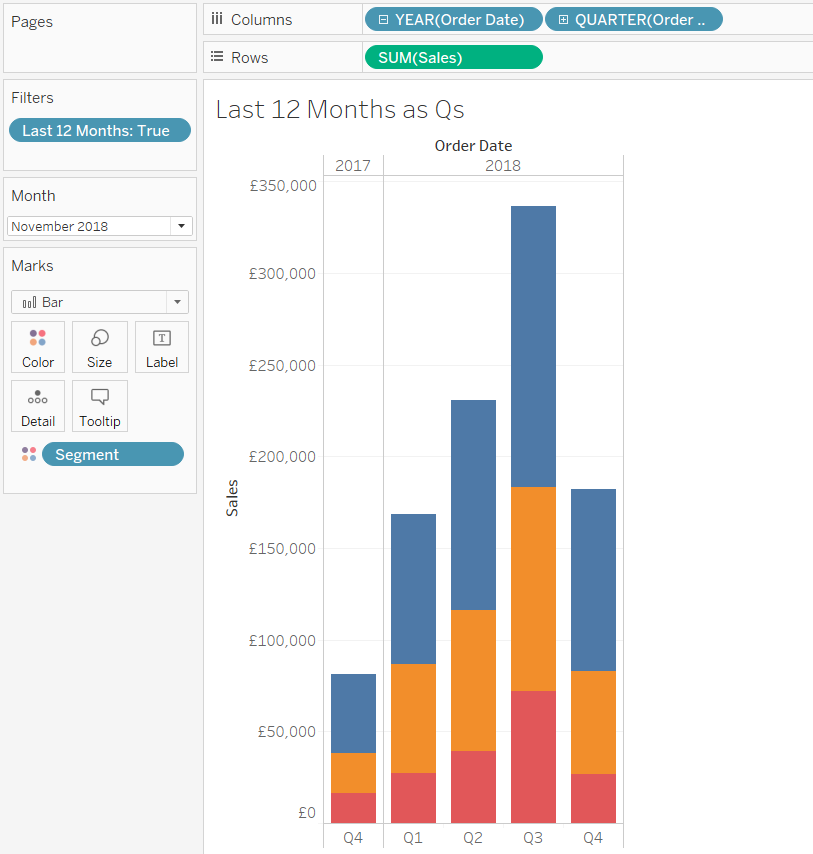Hold up a second, that doesn’t look right… let’s compare it with the unfiltered data: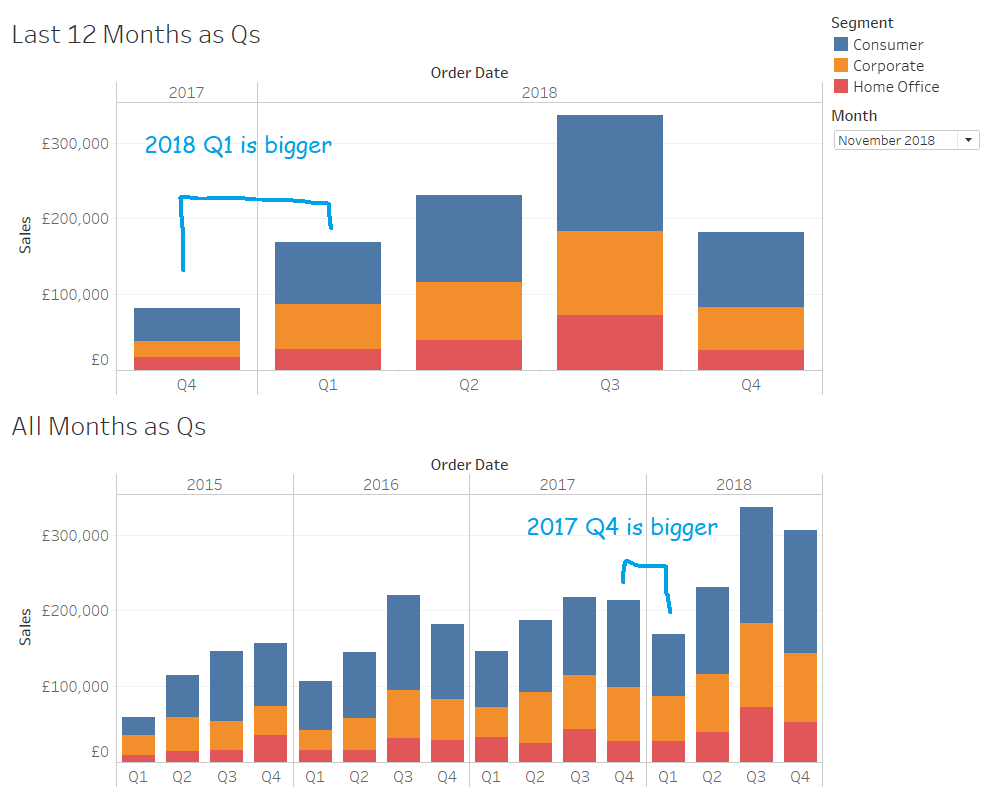What’s happened is that the Last Twelve Months filter has worked exactly as it’s supposed to; it’s filtered the data from 1st December 2017 to 30th November 2018. But the problem with that is that 2017 Q4 only has one month of data in it, and unless you stop to think about it, you could be misled into thinking that there really were more sales in 2018 Q1 than 2017 Q4.

Instead of filtering to exactly the last twelve months, we could filter to however many months it takes to show the current quarter and the previous three full quarters. So, if that’s November 2018, that would be October and November 2018 in the current quarter, and the three full quarters before that, from January 2018 to September 2018. If you select October 2018, it’d just be October 2018 in the current quarter, and then also from January 2018 to September 2018 for the three quarters before that.

This is where the modulo function comes in. You can create an if statement to change the number of months to filter to depending on how far through the quarter the selected month is. The modulo function returns the remainder left over when you divide by a particular number, so for our purpose, we can use the modulo function with the month number to work out how far through a quarter that month is. There are three months in a quarter, so we can use the month number % 3 to work out what the remainder is when we divide the month number by three. This will return the same number for each month at the same stage of a quarter.

For example, the months at the end of a quarter are March (3), June (6), September (9), and December (12). These all divide neatly by three, which means that Month Number % 3 is always going to be zero for the month sat the end of a quarter. The months in the middle of a quarter – February (2), May (5), August (8), and November (11) – don’t divide neatly by three, but they do all return a remainder of two when you calculate Month Number % 3. This calculation is a neat way of isolating how far through a quarter a month is, and you can see how it works in this table: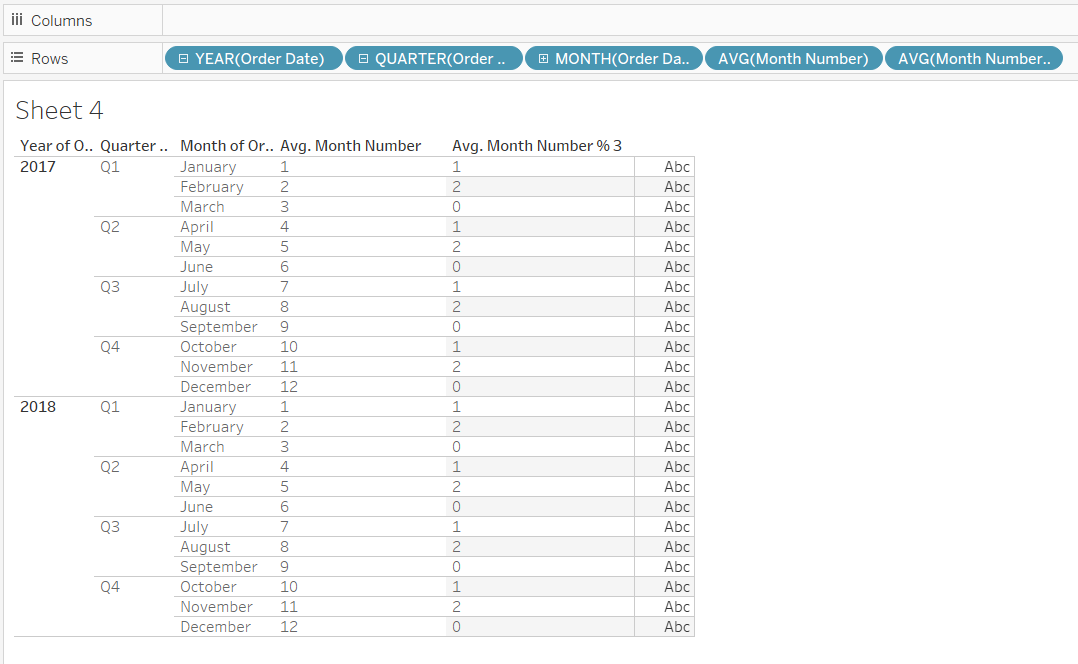We can use these remainders – 1, 2, and 0 for the first, second, and third months of a quarter – to create a filter with an if statement: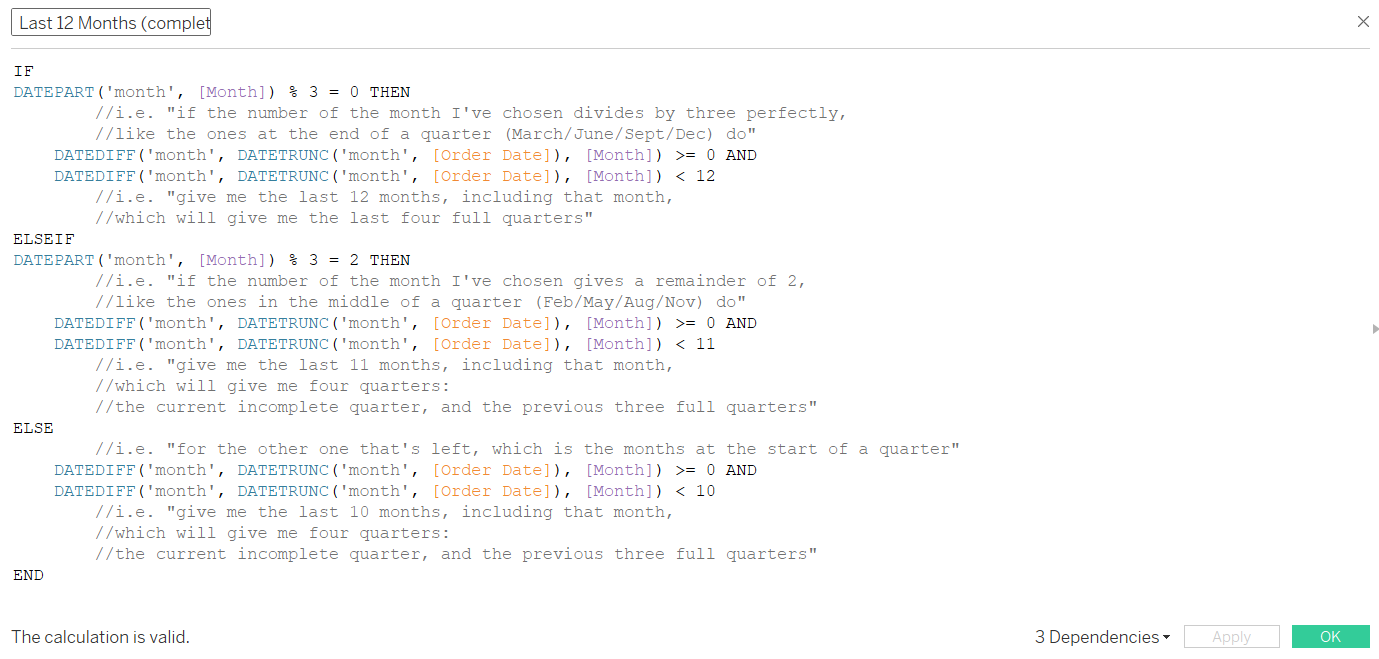That might be a little small to read properly, so here it is in text for you to copy/paste:

IF
DATEPART(‘month’, [Month]) % 3 = 0 THEN
DATEDIFF(‘month’, DATETRUNC(‘month’, [Order Date]), [Month]) >= 0 AND
DATEDIFF(‘month’, DATETRUNC(‘month’, [Order Date]), [Month]) < 12
ELSEIF
DATEPART(‘month’, [Month]) % 3 = 2 THEN
DATEDIFF(‘month’, DATETRUNC(‘month’, [Order Date]), [Month]) >= 0 AND
DATEDIFF(‘month’, DATETRUNC(‘month’, [Order Date]), [Month]) < 11
ELSE
DATEDIFF(‘month’, DATETRUNC(‘month’, [Order Date]), [Month]) >= 0 AND
DATEDIFF(‘month’, DATETRUNC(‘month’, [Order Date]), [Month]) < 10
END

Put that filter on all relevant worksheets and set it to TRUE, and sure enough, we get the current incomplete quarter (or the current complete quarter if you select March, June, September, or December), and the previous three full quarters: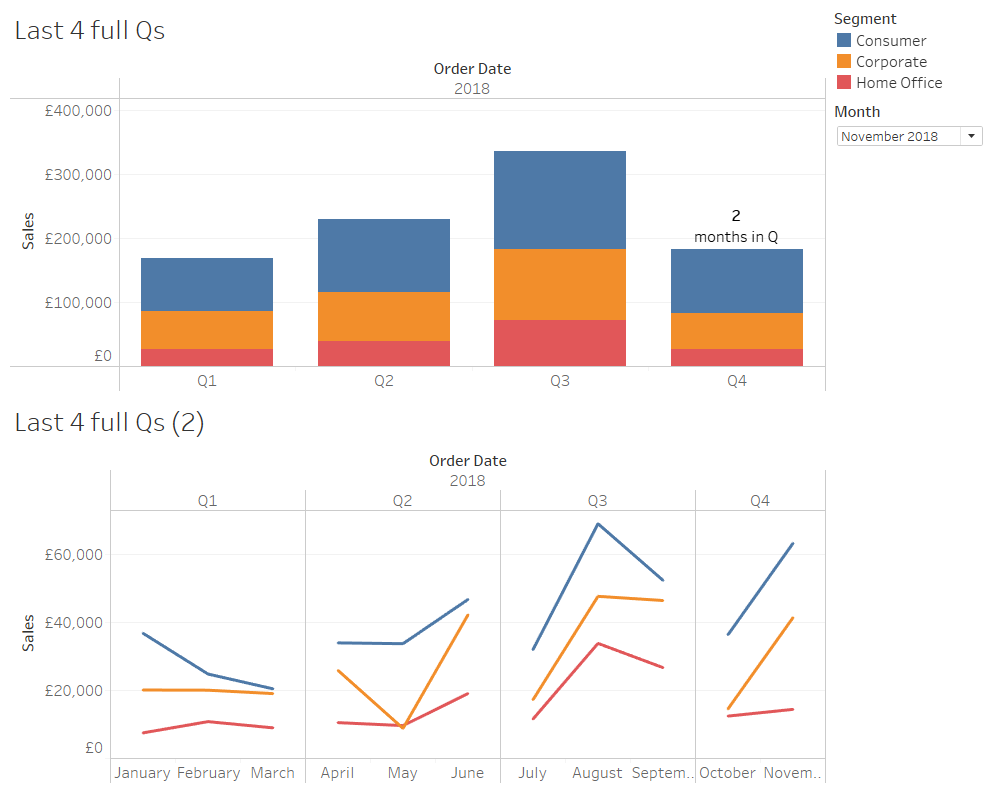This means you can see full quarters within a custom time range without seeing misleading quarterly data, and without using table calculations or LODs.

Standard# Language. Sex. Violins. Other?: how to create Violin Plots in Tableau.

Are you tired of histograms? Do you look at the count distribution of your actual data points and find yourself thinking, yeah, that’s cool and all, but I wish there was a more abstract way of showing this? Then you’ll probably like violin plots. That’s these things here: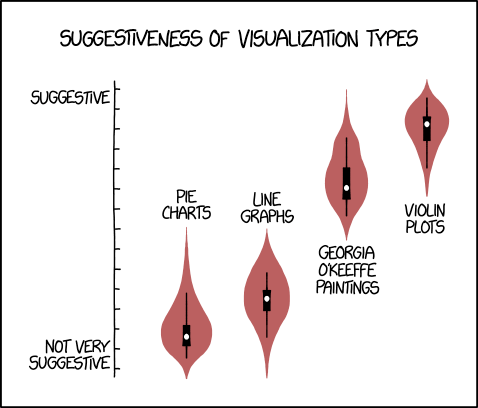Despite their somewhat sexual connotations, violin plots can be really useful for comparing distributions of data. To be honest, if it mattered that much to me, I’d probably go for a boxplot with overlaid, mostly transparent data points… but hey, people still use these, Tableau doesn’t support them natively, and I haven’t found a full tutorial anywhere (apologies if I’ve missed one – let me know!), so here’s how to make them.

To follow along, you can download the Tableau workbook I used from my Tableau Public page here.

It’s all based around Kernel density estimation. This is maths for “take my data, smooth it out a bit, and make it so I can generalise it to data I haven’t got yet”. You can read more about that here, and I’m going to use the same set of six values used in the Wikipedia example.

Here’s what you’ll need, and here’s one I made earlier:

1. Your data. One column with one row per observation, one column with one row per observation ID. Something a little like this:2. A handy data scaffold. I’ve used a hundred points, going from zero to 99; if your data has a lot of variance, you might want to whack that up to a thousand, although that’ll make things proper slow. Either way, keep it simple; it should look like this:Okay, nice. Stick these into Tableau, and join them with a custom join calculation so that every row in the data joins to every row in the scaffold (i.e. six rows balloons out to 600 rows here; this is why using a 1000 row scaffold isn’t pretty, performance-wise). I normally just type in “join” on both sides:Also, remember that with a scaffolded dataset, simply summing your values will just multiply the value you actually want by a hundred. Watch out for that.

Okay, we’ve got our data; let’s plot the sample values we want to create a violin plot of.What we need to do is draw a kernel around each data point, like this (but better):…and add up the y-axis values of those kernels to create the overall kernel density, like this (but a lot better):This is why we need the data scaffold; you can’t draw a kernel with one point, so we need a hundred points for each point.

The first thing to do is to create an adjusted x-axis. We want the hundred points for each data point to range from the lowest to the highest value. You can do that like this (ignore the bandwidth part for now):

```IF [X] = 0 THEN {MIN([Sample Value])} - [X scaling factor] ELSEIF [X] = 99 THEN {MAX([Sample Value])} + [X scaling factor] ELSE ({MIN([Sample Value])} - [X scaling factor]) + ( ABS( ({MAX([Sample Value])}+[X scaling factor]) - ({MIN([Sample Value])}-[X scaling factor]) ) * ([X]/99) ) END```

Alternatively, you can see that there’s no point making the scaffolded points for the values go all the way across the range, so you could fix it on the Sample ID instead. But I found that this had a knock-on effect down the line that I didn’t like, so let’s leave this for now. If you can make it work, I’d love to hear from you.

We’ve now got a set of Adjusted X data points across the range of the data for each data point:The next step is to stick something on the y-axis so that each point goes up the required amount to draw a kernel around each data point. It’ll end up looking like this:…and the calculation required to do that is this:

```1/({COUNTD([Sample ID])}*[bandwidth (wiki example)]) * (1/(SQRT(2*PI()))) * EXP(-0.5 * ( ([Adjusted X] - [Sample Value])^2)/[bandwidth (wiki example)]) ```

This is done as a normal kernel using the standard normal density function, because that’ll probably do the job well enough for most situations. I’m not going to go into the different types of kernel functions, but you can read about them here, and if a different kernel function tickles your fancy, you can rewrite the (1/(SQRT(2*PI()))) * EXP(-0.5 * ( part of the equation with something else.

I’m also not going to go into bandwidths, because it’s complicated. There are various proper methods for choosing your bandwidth, but if you play about with it, you’ll see that setting the bandwidth too low doesn’t smooth out the curve enough, and setting the bandwidth too high smooths out the curve too much.Anyway. To create the kernel density estimation for the data points, we need to sum up the individual kernels. This is the easy part in Tableau; CTRL+drag the same kernel calculation field to rows again, take Sample ID off colour/detail, sum it up, and put it on a synchronised dual axis. Voilà.This grey curve is half a violin plot on its side. But before we go into how to rotate and fill it, let’s go back to the scaling factor. I’ve kept it at 0 the whole way through, so that the x-axis runs from the smallest data point to the highest data point. That’s fine if you’re showing your actual data, but the whole point of kernel density estimates is to show a probability function… or in other words, “okay this is the data I’ve got, but what if there’s going to be more data like this, where’s it going to go?”. There may well be other values higher than your highest point or lower than your lowest point. So, I created a parameter to mess about with how far the x-axis goes, simply by adding a constant to the highest value and subtracting that same constant from the lowest value. You can adjust it as you see fit; I think setting it to 4 captures this data nicely:Right. That’s the maths behind a violin plot. Now to actually make one.

All we need to do is fill it and rotate it. The filling is easy; just convert it from line to area:…but the rotation messes this right up.So, we need to redraw it as a polygon. And to do that, we need to redo some of the calculations. Sorry about that.

Firstly, make this change to the Adjusted X calculation:

```IF [X] = 0 THEN ({MIN([Sample Value])} - [X scaling factor]) ELSEIF [X] = 1 THEN ({MIN([Sample Value])} - [X scaling factor]) ELSEIF [X] = 99 THEN ({MAX([Sample Value])} + [X scaling factor]) ELSE ({MIN([Sample Value])} - [X scaling factor]) + ( ABS( ({MAX([Sample Value])}+[X scaling factor]) - ({MIN([Sample Value])}-[X scaling factor]) ) * (([X]-1)/97) ) END ```
And now make this change to your kernel calculation:

```IF [X] = 0 THEN 0 ELSEIF [X] = 99 THEN 0 ELSE 1/({COUNTD([Sample ID])}*[bandwidth (wiki example)]) * (1/(SQRT(2*PI()))) * EXP(-0.5 * ( ([Adjusted X (polygon)] - [Sample Value])^2)/[bandwidth (wiki example)]) END ```

That should do the trick. If you’re using a bigger scaffold, remember to update the 99 to 999 and the 97 to 997! Now you can plot your polygon like this:And if you repeat the kernel calculation, whack a minus on the front of it, and dual axis it, you can make a nice violin:These violins take a lot of formatting to make, and it’s an absolute faff to compare two separate distributions. And the LODs for finding the max and min values in the data will require you to add in a FIXED for any dimension you want in the view. They’ll also screw up filters, unless you put them in context. It is possible, though; here’s an unformatted set of violins for Sales in each Category in California using Tableau’s Superstore dataset. With some a fair bit of tidying, this could look pretty good:Again, it’s not an ideal way of showing the distributions, and hopefully Tableau introduce violin plots in the same way as boxplots in a later version. But for now, this is how you’d do it if you really wanted one.

Standard# We chase the waves: how to make a sinusoidal time series in Tableau.

Browsing what other people have done on Tableau Public is a great source of both challenge and inspiration. Recently, I’ve been really taken with Neil Richards’ visualisation of football league winners over time, with a beautiful sine wave showing how long it’s been since each team last won the league. I’ve no idea what to call these plots, but they’re fantastic (click image to see Neil’s original on Tableau Public).I’ve wanted to take these apart and see how they work for a while, and finally got round to it the other day. It turns out that Neil did a lot of the angle calculations outside Tableau, which is fair enough… so I set myself the challenge of doing it all with table calculations. I got there eventually, but it was a good workout.

This blog is a walk-through of how to do it. Instead of football data, I’ve used official pope names; I was on a wikipedia spiral and noticed that seven of the eleven popes between 1775 and 1958 were called Pius, taking the Pius count from VI to XII. Naturally this reminded me of Barcelona’s recent league dominance, winning six of the last nine La Liga championships, so it seemed obvious to see if the chart for pope names would be similarly tightly woven.

So. This is all the input data you’ll need: a list of all popes, in order, with a record ID and a number showing how many of that name there’s been so far:Come to think of it, if you’re good with INDEX() calcs, you might not even need the PopeNameNo column… but I’m not, so I do.

You’ll also need a simple scaffold sheet with 100 points, going from 0 to 99. If you’re trying to visualise something with more data points than the 267 popes I’ve got, you might want to whack up the scaffold to 999 instead.[I’m including the elected-but-not-consecrated Stephen II in this list, because even though he’s not an official pope, all the subsequent Stephens had an increased number until relatively recently, and then it got confusing. So he’s in here.]

Read the two files into Tableau, and create a calculated join with “x” in the join field for the popes data, so that there are 100 points for each pope. Now we’re ready to do some vizzical jiggery-popery!

Although Neil’s vizzes had time on a y-axis going vertically, I’ve spent way too long looking at time series graphs for that to feel intuitive, so I’m reverting to the vanilla “time on x-axis going left” approach.  Let’s stick Pope ID on the x-axis as a continuous dimension:Great, we’ve got a line made up of lots of circles. This doesn’t make it easy to see what’s going on, so let’s filter to a single pope name – Leo will do for now:Here’s all the Leos, in order. It was a fairly popular (pope-ular?) pope name in the later part of the first millenium, but then it fell out of favour for a while, with almost 500 years between Leo IX in 1054 and Leo X in 1513.

We want to connect these dots with a line, but if we set the mark type to line, it’ll just be a straight line. Rather, we want a curved line, like this:This is why we’ve got the scaffold table. We can’t just connect two points with a curvy line – or at least, I can’t. Instead, we need to put a load of dots between the two main points, and connect them up. That means figuring out the x and y axis values to put those dots in the right place to connect the two main points with a nice sine wave.

To do that, we’ll need to create a new x-axis measure instead of simply Pope ID, where the distance (in units of popes) is divided by up so that the scaffold points are evenly distributed along the x-axis. But first, that means calculating the distance between popes in units of popes. We can do that with a lookup() calculation:

`LOOKUP(ATTR([Pope ID]), 1)`This is working nicely – I’ve stuck it on the tooltip, and hovering over Leo IX, who’s pope number 153 in my list, tells me that the next Leo is pope number 218.

This’ll work fine for this filtered view, but to get it to do this properly, you’ll need to put the Point field from the scaffold table on detail, and edit the table calculation to compute using Point and Pope ID:At the moment, all those points are on top of each other on the Pope ID value. This isn’t what we want – we want to spread them out evenly between the Pope ID values. To do that, we’ll need this calculation here. It’s a bit long, and there’s MIN() functions everywhere because of all the table calculations, but hey:Logically, what it’s doing is this:

1. There are 100 scaffolding points, going from 0 to 99.
2. If it’s the first one, i.e. 0, give it the same value as Pope ID. For the Leo IX to Leo X example, this is 153.
3. If it’s the last one, give it the same value as the next Pope ID with the same name. For the Leo IX to Leo X example, this is 218.
4. If it’s any of the rest, calculate the difference between the two Pope ID values (i.e. 218 – 153, which is 65 pope units), and then divide that by the maximum point value, which is 99 (if you made your scaffold points 1-100 instead, you’ll have to set this to maximum point value -1, not 100). This is because there’s 99 spaces to fill between all the scaffold points. Then multiply that fraction by the number of the point, and add it to the Pope ID value.

You can also copy and paste it directly from here if that makes it easier:

```IF MIN([Point]) = 0 THEN MIN([Pope ID]) ELSEIF MIN([Point]) = MIN([MaxPoint]) THEN [NextPopeID] ELSE MIN([Pope ID]) + ( ([NextPopeID] - MIN([Pope ID])) / MIN([MaxPoint]) * MIN([Point]) ) END```

Grand. Set the new x-axis value to calculate using Pope ID and Point, restarting every Pope ID, and that’s the x-axis sorted. But these points are still basically just calculating a straight line, whereas what we actually need to do is push them up the y-axis by a different amount, kind of like this:Let’s also add a direction calculation, so that the wave between the first and second goes upwards, the wave between the second and third goes downwards, and so on. We can do that by working out whether it’s an odd or even number, and setting the direction accordingly:

`IF INT([Pope Name No] % 2) = 0 THEN -1 ELSE 1 END`

Now let’s work on our y-axis calculation. It’s got three parts:

1. Working out a nice sinusoidal curve
2. Multiplying that value by how long it’s been between popes, so that the longer it is between popes, the higher the curve goes
3. Multiplying that by the distance calculation so that it goes above or below the x-axis accordingly

The first phase of a sine wave goes from 0 on the y-axis, up to a peak of 1, and then back down to 0 between the x-axis values of 0 and π, like so:In our case, we don’t want a wave between 0 pope units and 3.141… pope units; rather, we want to define the beginning and end of this phase of a sine wave to be between one pope and the next pope of the same name. So, for Leo IX to Leo X, we want 153 to be our 0 and 218 to be our π. That means taking the scaffold point, dividing it by the maximum point of 99 to get the % of the distance that that point is along the 0-to-π scale, and then multiply it by π.

That’ll give us the first phase of a sine wave of the same height (of 1) between the popes, regardless of how long it’s been between them. We want the peak to be higher the longer it’s been between popes, so we multiply it by the distance. Then we can multiply by our positive/negative direction calc. Here’s the code:

```MIN(SIN([Point]/[MaxPoint] * PI())) * ([NextPopeID] - MIN([Pope ID])) * MIN([Direction])```

So, stick the y-axis calc on rows, set the table calculation to calculate using Pope and Pope ID, and voila! We have a nice set of sine waves between our Leos.(this plot reminds me of doing Fourier transformations for EEG analysis; technically, we haven’t created this complex wave by layering up different sine waves on top of each other, but we can kind of decompose it into sets of individual sine waves as we go along)

The hard work is done now, so let’s bring the rest of our popes back in:Delightful. The rest of it is all about making it pretty, which I can leave to your personal tastes. But the real question is: what happens with Pius, the Barcelona of second millenium popes? Can we clearly see the era of Pius dominance?…yes, we can.

These graphs can be applied to basically anything that goes in a sequential order and may or may not have repeated values; this graph here is every word from my old band’s EP in order. I like how you can see where the choruses are, because the lines get more tightly woven as the words in the chorus are repeated more often.I hope this blog makes it clear how to make these graphs! I still don’t know what to call them, but in my head they’re unimaginatively down as sine wave time series. Thanks again to Neil for creating them first, and for making his workbooks downloadable and play-around-withable!

Standard